NEET  >  31 Years NEET Previous Year Questions: Solutions - 2

# 31 Years NEET Previous Year Questions: Solutions - 2

Test Description

## 30 Questions MCQ Test Chemistry Class 12 | 31 Years NEET Previous Year Questions: Solutions - 2

31 Years NEET Previous Year Questions: Solutions - 2 for NEET 2022 is part of Chemistry Class 12 preparation. The 31 Years NEET Previous Year Questions: Solutions - 2 questions and answers have been prepared according to the NEET exam syllabus.The 31 Years NEET Previous Year Questions: Solutions - 2 MCQs are made for NEET 2022 Exam. Find important definitions, questions, notes, meanings, examples, exercises, MCQs and online tests for 31 Years NEET Previous Year Questions: Solutions - 2 below.
Solutions of 31 Years NEET Previous Year Questions: Solutions - 2 questions in English are available as part of our Chemistry Class 12 for NEET & 31 Years NEET Previous Year Questions: Solutions - 2 solutions in Hindi for Chemistry Class 12 course. Download more important topics, notes, lectures and mock test series for NEET Exam by signing up for free. Attempt 31 Years NEET Previous Year Questions: Solutions - 2 | 30 questions in 60 minutes | Mock test for NEET preparation | Free important questions MCQ to study Chemistry Class 12 for NEET Exam | Download free PDF with solutions
 1 Crore+ students have signed up on EduRev. Have you?
31 Years NEET Previous Year Questions: Solutions - 2 - Question 1

### An ideal solution is formed when its components 

Detailed Solution for 31 Years NEET Previous Year Questions: Solutions - 2 - Question 1

For ideal solution,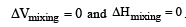31 Years NEET Previous Year Questions: Solutions - 2 - Question 2

### All form ideal solution except 

Detailed Solution for 31 Years NEET Previous Year Questions: Solutions - 2 - Question 2

C2H5I and C2H5OH form non-ideal solution.

31 Years NEET Previous Year Questions: Solutions - 2 - Question 3

### The relative lowering of the vapour pressure is equal to the ratio between the number of 

Detailed Solution for 31 Years NEET Previous Year Questions: Solutions - 2 - Question 3

According to Raoult's law, the relative lowering in vapour pressure of a dilute solution is equal to the mole fraction of the solute present in the solution.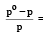mole fraction of solute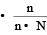31 Years NEET Previous Year Questions: Solutions - 2 - Question 4

Which of the following aqueous solution has minimum freezing point ? 

Detailed Solution for 31 Years NEET Previous Year Questions: Solutions - 2 - Question 4

ΔTf = i × Kf × m Van't Hoff factor, i = 2 for NaCl, hence ΔTf = 0.02 Kf which is maximum in the present case.
Hence ΔTf is maximum or freezing point is minimum.

31 Years NEET Previous Year Questions: Solutions - 2 - Question 5

Blood cells retain their normal shape in solution which are 

Detailed Solution for 31 Years NEET Previous Year Questions: Solutions - 2 - Question 5

Blood cells neither swell n or shrink in isotonic solution. As isotonic solutions have equal concentration therefore there is no flow of solvent is found and hence solvent neither enters nor flow out of the blood cells.

31 Years NEET Previous Year Questions: Solutions - 2 - Question 6

Which of the following modes of expressing concentration is independent of temperature ? [1992,1995]

Detailed Solution for 31 Years NEET Previous Year Questions: Solutions - 2 - Question 6

The molality involves weights of the solute and the solvent. Since weight does not change with the temperature, therefore molality does not depend upon the temperature.

31 Years NEET Previous Year Questions: Solutions - 2 - Question 7

Which one is a colligative property ? 

Detailed Solution for 31 Years NEET Previous Year Questions: Solutions - 2 - Question 7

Osmotic pressure is a colligative property.

31 Years NEET Previous Year Questions: Solutions - 2 - Question 8

If 0.1 M solution of glucose and 0.1 M solution of urea are placed on two sides of the semipermeable membrane to equal heights, then it will be correct to say that 

Detailed Solution for 31 Years NEET Previous Year Questions: Solutions - 2 - Question 8

As both the solutions are isotonic hence there is no net movement of the solvent through the semipermeable membrane between two solutions.

31 Years NEET Previous Year Questions: Solutions - 2 - Question 9

At 25°C, the highest osmotic pressure is exhibited by 0.1 M solution of 

Detailed Solution for 31 Years NEET Previous Year Questions: Solutions - 2 - Question 9

Conc. of particles in CaCl2 sol. will be max. as i = 3 is max.
Note : Glucose and Urea do not dissociate into ions, as they are nonelectrolytes.

31 Years NEET Previous Year Questions: Solutions - 2 - Question 10

Which one of the following salts will have the same value of van’t Hoff factor (i) as that of K4[Fe (CN)6]. 

Detailed Solution for 31 Years NEET Previous Year Questions: Solutions - 2 - Question 10

K4 [Fe(CN)6] and Al2(SO4)3 both dissociates to give 5 ions or i = 5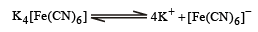and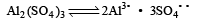31 Years NEET Previous Year Questions: Solutions - 2 - Question 11

The number of moles of oxygen in one litre of air containing 21% oxygen by volume, in standard conditions, is 

Detailed Solution for 31 Years NEET Previous Year Questions: Solutions - 2 - Question 11

Percentage volume of oxygen = 21%. (Given)
∵ 100 ml of air contains = 21ml of O2
∴ Volume of oxygen in one litre of air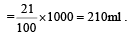Therefore no. of moles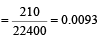(∵ volume of 1 litre of gas at S.T.P. is 22400 ml)

31 Years NEET Previous Year Questions: Solutions - 2 - Question 12

Vapour pressure of benzene at 30°C is 121.8 mm.When 15 g of a non volatile solute is dissolved in 250 g of benzene its vapour pressure decreased to 120.2 mm. The molecular weight of the solute (Mo. wt. of solvent = 78) 

Detailed Solution for 31 Years NEET Previous Year Questions: Solutions - 2 - Question 12

Give vapour pressure of  pur e solute (P0) = 121.8 mm;
Weight of solute (w) = 15 g
Weight of solvent (W) = 250 g;
Vapour pressure of pure solvent (P) = 120.2 mm and Molecular weight of solvent (M) = 78 From Raoult’s law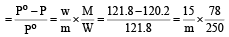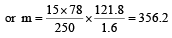31 Years NEET Previous Year Questions: Solutions - 2 - Question 13

According to Raoult's law, relative lowering of vapour pressure for a solution is equal to  [1995

Detailed Solution for 31 Years NEET Previous Year Questions: Solutions - 2 - Question 13

Relative lowering of vapour pressure depends upon the mole fraction of solute.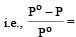mole fraction of solute

31 Years NEET Previous Year Questions: Solutions - 2 - Question 14

The vapour pressure at a given temperature of an ideal solution containing 0.2 mol of a nonvolatile solute and 0.8 mol of solvent is 60 mm of Hg. The vapour pressure of the pure solvent at the same temperature is 

Detailed Solution for 31 Years NEET Previous Year Questions: Solutions - 2 - Question 14

According to Raoult's law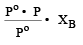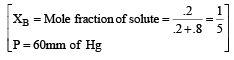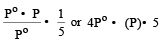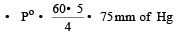31 Years NEET Previous Year Questions: Solutions - 2 - Question 15

Which of the following 0.10 m aqueous solutions will have the lowest freezing point ? 

Detailed Solution for 31 Years NEET Previous Year Questions: Solutions - 2 - Question 15

Depression in F.P. ∝ No. of particles.
Al2(SO4)3 provides five ions on ionisation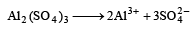while KCl provides two ions
KCl     K    Cl
C6H12O6 and C12H22O11 are not ionised so they have single particle.
Hence, Al2(SO4)3 have maximum value of depression in F.P or lowest F.P

31 Years NEET Previous Year Questions: Solutions - 2 - Question 16

A 5% solution of cane sugar (mol. wt. =342) is isotonic with 1% solution of a substance X. The molecular weight of X is 

Detailed Solution for 31 Years NEET Previous Year Questions: Solutions - 2 - Question 16

Osmotic pressure of 5% cane sugar solution 5% cane sugar solution means 100ml of solution contain cane sugar = 5g

∴    1000 of solution contain cane sugar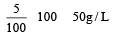Similarily 1% is solution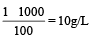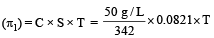Osmotic pressure of 1% solution of susbtance  (π2)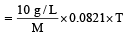As both are isotonic, So
π1 = π2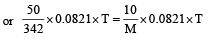∴  M (mol. wt. of X)31 Years NEET Previous Year Questions: Solutions - 2 - Question 17

The vapour pressure of a solvent decreased by 10mm of mercury when a non-volatile solute was added to the solvent. The mole fraction of the solute in the solution is 0.2. What should be the mole fraction of the solvent if the decrease in the vapour pressure is to be 20mm of mercury?

Detailed Solution for 31 Years NEET Previous Year Questions: Solutions - 2 - Question 17

According to Raoult's law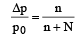(mole fraction of solute)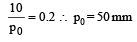For other solution of same solvent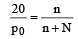(Mole fraction of solute)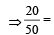mole fraction of solute

⇒   Mole fraction of solute = 0.4 As mole praction of solute + mole traction of solvent = 1

Hence, mole fraction of solvent = 1 – 0.4    = 0.6

31 Years NEET Previous Year Questions: Solutions - 2 - Question 18

Which of the following statements, regarding the mole fraction (x) of a component in solution, is incorrect? 

Detailed Solution for 31 Years NEET Previous Year Questions: Solutions - 2 - Question 18

Mole fraction of any component A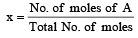As total no. of moles > no. of moles of A thus x can never be equal to one on zero.

31 Years NEET Previous Year Questions: Solutions - 2 - Question 19

Which of the following colligative property can provide molar mass of proteins (or polymers or colloids) with greatest precision ? 

Detailed Solution for 31 Years NEET Previous Year Questions: Solutions - 2 - Question 19

Molecular masses of polymers are best determined by osmotic pressure method .
Firstly because other colligative properties give so low values that they cannot be measured accurately and secondly, osmotic pressure measurements can be made at room temperature and do not require heating which may change the nature of the polymer.

31 Years NEET Previous Year Questions: Solutions - 2 - Question 20

The beans are cooked earlier in pressure cooker, because 

Detailed Solution for 31 Years NEET Previous Year Questions: Solutions - 2 - Question 20

The beans are cooked earlier in pressure cooker because boiling point increases with increasing pressure.

31 Years NEET Previous Year Questions: Solutions - 2 - Question 21

Molarity of liquid HCl will be, if density of solution is 1.17 gm/cc 

Detailed Solution for 31 Years NEET Previous Year Questions: Solutions - 2 - Question 21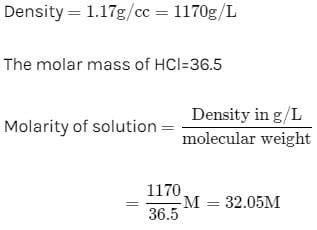31 Years NEET Previous Year Questions: Solutions - 2 - Question 22

1 M, 2.5 litre NaOH solution is mixed with another 0.5 M, 3 litre NaOH solution. Then find out the molarity of resultant solution 

Detailed Solution for 31 Years NEET Previous Year Questions: Solutions - 2 - Question 22

From molarity equation M1V1 + M2V2 = MV
1× 2.5 + 0.5 × 3 = M × 5.5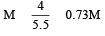31 Years NEET Previous Year Questions: Solutions - 2 - Question 23

A solution con tains non -volatile solute of molecular mass M2. Which of the following can be used to calculate the molecular mass of solute in terms of osmotic pressure ? 

Detailed Solution for 31 Years NEET Previous Year Questions: Solutions - 2 - Question 23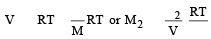31 Years NEET Previous Year Questions: Solutions - 2 - Question 24

A solution containing components A and B follows Raoult's law when 

Detailed Solution for 31 Years NEET Previous Year Questions: Solutions - 2 - Question 24

These two components A and B follows the condition of Raoult’s law if the force of attraction between A and B is equal to the force of attraction between A and A or B and B.

31 Years NEET Previous Year Questions: Solutions - 2 - Question 25

Formation of a solution from two components can be considered as 
(i) Pure solvent → separated solvent                              molecules, ΔH1
(ii) Pure solute   → separated solute                               molecules, ΔH2
(iii) Separted solvent & solute molecules → Solution,  ΔH3
Solution so formed will be ideal if

(a) ΔHsoln = ΔH1 + ΔH2 - ΔH3

(b) ΔHsoln = ΔH1 + ΔH2 + ΔH3

(c) ΔHsoln = ΔH1 - ΔH2 - ΔH​​​​​​​3

(d) ΔHsoln = ΔH3 - ΔH1 - ΔH​​​​​​​2

Detailed Solution for 31 Years NEET Previous Year Questions: Solutions - 2 - Question 25

For an ideal solution, ΔHmixing = 0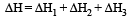(Accroding to Hess's law) i.e., for ideal solutions there is no change in magnitude of the attractive forces in the two components present.

31 Years NEET Previous Year Questions: Solutions - 2 - Question 26

Camphoris often used in molecular mass determination because 

Detailed Solution for 31 Years NEET Previous Year Questions: Solutions - 2 - Question 26

Solvent having high cryoscopic constant can be used in determination of molecular mass by cryoscopic method.

31 Years NEET Previous Year Questions: Solutions - 2 - Question 27

The vapour pressure of two liquids ‘P’ an d ‘Q’ are 80 and 60 torr, respectively. The total vapour pressure of solution obtained by mixing 3 mole of P and 2 mole of Q would be 

Detailed Solution for 31 Years NEET Previous Year Questions: Solutions - 2 - Question 27

Given V.PP = 80 torr  V.PQ = 60 torr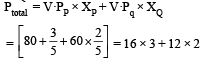Ptotal = 48 + 24 = 72 torr

31 Years NEET Previous Year Questions: Solutions - 2 - Question 28

A solution of urea (m ol. mass 56 g mol-1) boils at 100.18°C at the atmospheric pressure. If Kf and Kb for water are 1.86 and 0.512 K kg mol-1 respectively, the above solution will freeze at 

Detailed Solution for 31 Years NEET Previous Year Questions: Solutions - 2 - Question 28

As ΔTf = Kf. m
ΔTb = Kb. m

Hence, we have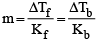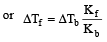= [ΔTb = 100.18 - 100 = 0.18°C]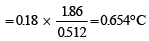As the Freezing Point of pure water is 0°C,
ΔTf = 0 –Tf
0.654 = 0 – Tf
∴  Tf = – 0.654 thus the freezing point of solution will be – 0.654°C.

31 Years NEET Previous Year Questions: Solutions - 2 - Question 29

The mole fraction of the solute in one molal aqueous solution is: [2 00 5]

Detailed Solution for 31 Years NEET Previous Year Questions: Solutions - 2 - Question 29

One molal solution means one mole solute present in 1 kg (1000 g) solvent i.e., mole of solute = 1

Mole of solvent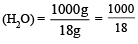Mole fraction of solute  =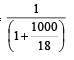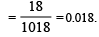31 Years NEET Previous Year Questions: Solutions - 2 - Question 30

A solution of acetone in ethanol 

Detailed Solution for 31 Years NEET Previous Year Questions: Solutions - 2 - Question 30

A solution of acetone in ethanol shows positive deviation from Raoult's law. It is because ethanol molecules are strongly hydrogen bonded. When acetone is added, these molecules break the hydrogen bonds and ethanol becomes more volatile. Therefore its vapour pressure is increased.

## Chemistry Class 12

157 videos|362 docs|261 tests
 Use Code STAYHOME200 and get INR 200 additional OFF Use Coupon Code
Information about 31 Years NEET Previous Year Questions: Solutions - 2 Page
In this test you can find the Exam questions for 31 Years NEET Previous Year Questions: Solutions - 2 solved & explained in the simplest way possible. Besides giving Questions and answers for 31 Years NEET Previous Year Questions: Solutions - 2, EduRev gives you an ample number of Online tests for practice

## Chemistry Class 12

157 videos|362 docs|261 tests

### How to Prepare for NEET

Read our guide to prepare for NEET which is created by Toppers & the best Teachers